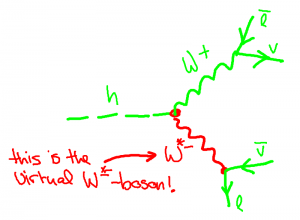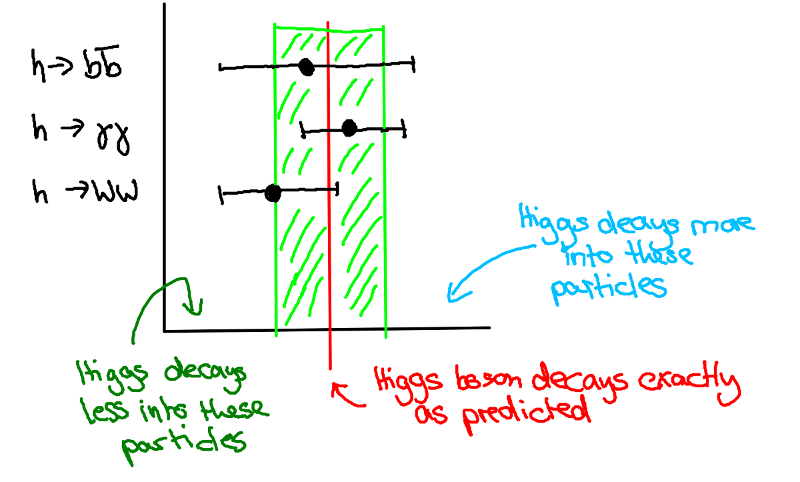Categories
Pages
-A Higgs or not a Higgs?

July 12th, 2014 | byIt has been exactly two years now since the two big experiments Atlas and CMS at the LHC announced the discovery of a new boson. This boson is so far hotly tipped to be the Standard Model Higgs particle. What does “being the Standard model Higgs particle” mean, are particle physicists sure about that and how do they come to their conclusion?

The Higgs particle is a result of the so-called Higgs mechanism which is incorporated in the Standard model of particle physics. This theory describes all the interactions of the different elementary particles, and the Higgs mechanism is one part of it. It is needed to describe a phenomenon that is called electroweak symmetry breaking which is closely linked to the masses of the elementary particles. The Higgs particle is so far the only elementary particle which has spin 0. Particles with integer spin are bosons and therefore it is called Higgs boson.

However, I don’t want to describe the Higgs mechanism here, since we want to do this another time in more detail. Instead, let us have a look at how the Higgs particle is actually produced at the LHC and how its properties can be determined!

At the Large Hadron Collider protons are accelerated to a speed of 99.999997% of the velocity of light, and are smashed together at several interaction points in the middle of detectors. Two of them, Atlas and CMS record what is important for us then:

With a certain probability, two of the protons interact and, if the energy is high enough, a Higgs particle is produced, again with a certain probability. This particle is unstable, which means that its lifetime (roughly 10^-22 seconds) is extremely short and it decays into other (lighter) particles. The energy and momentum of those can then be measured by the detectors Atlas and CMS:The mass of the Higgs boson was measured with the help of two specific decays, in which all decay products can be seen in the detector. If you add up all the energy of the decay products, you get the Higgs bosons’ mass. As you might have heard, it is measured to roughly 125 GeV.

The nice thing about the Standard Model Higgs boson is that, once the mass is known, all its properties are predicted by the Higgs mechanism. This makes it possible to test this theory in detail and conclude whether the observed particle is the actual Standard model Higgs boson or another particle that just looks like the Higgs boson at the first glimpse.

One important ingredient is that the interaction strength of the Higgs boson to elementary particles is proportional to the mass of these particles. The heavier the particle is, the larger is the interaction probability of the Higgs boson with this particle.

That has an important impact on the decays of the Higgs particle: The Higgs boson decays mostly into those particle that are the heaviest!  Of course, we have to consider energy conservation at this point. The masses of the decay products have to come from the mass of the Higgs boson and thus the sum has to be equal or smaller then the Higgs boson mass. If the Higgs boson had the mass of 100 GeV, it just cannot decay into two (equal) particles that are heavier than 50 GeV.

Before the mass was known, one could merely calculate how often and into which particles the Higgs decays depending on its mass:The original plot with even more lines can be found here. What I have sketched here are the so called “branching ratios”. They give the probability that a Higgs boson decays into a specific particle pair. Since the interactions strength is proportional to the mass of the interaction partners, the Higgs boson really wants to decay predominantly into the heaviest particle pairs that are energetically possible. If, hypothetically, the Higgs boson had a mass of 100 GeV, the predominant decay would be into a pair of bottom quarks. You can read this off the grey line in the sketch. Those quarks have a mass of roughly 4 GeV, so it is no problem for 100 GeV Higgs boson to provide this energy. The next heaviest particle pairs are the Tau leptons with roughly 1.8 GeV per tau lepton (marked with the light-green line).

This is of course not the whole story: Maybe you wanted to check this and have looked up (or know) the W boson mass. W bosons are very heavy particles with roughly 80 GeV. So, from what I said before, it is energetically not possible for a 100 GeV Higgs boson to decay into two W bosons. However, the dark green line above, marking the ratio of Higgses that decay into these W bosons, is already there below the 160 GeV Higgs mass. So what happens here? This is due to the fact that the Higgs boson can also decay into so-called virtual W bosons. Those virtual W bosons immediately decay further to their decay products. A virtual particle is one, where the connection between energy and mass as it is described in special relativity does not hold for a very short moment. But this is another topic and for the moment we will just accept this as a fact.

So, let’s go back to the bosonic particle that was discovered at the LHC. As already mentioned, the mass of this particle was determined to 125 GeV. So from the sketch above we can have a look at the particles it predominantly decays into. This is  the decay into bottom quarks with roughly 60%:and the decay into two W bosons (where one is “virtual”) kicks in with roughly 25%:(These funny diagrams is what one calls Feynman diagrams. One draws them for decays and other processes like these. Not only do they give a nice intuition, but also stand for mathematical expressions which allow you to calculate the probabilities for these decays or processes.)
Unfortunately, the decays of the Higgs bosons to bottom quarks are most frequent ones, but at the same time one of the hardest to measure. So one looks into decays which are simpler and cleaner to detect. One of these is the process of the Higgs boson decaying into two photons. I have marked them with the light blue color in the sketch above. You can see that the probability of a Higgs boson decaying into two photons is only 0.1%! Since the start of data recording at the LHC, roughly half a million Higgs bosons have been produced. From this amount, 0.1% have decayed into photons, so there have been roughly 500 of these high energetic photon pairs since 2009! So how come that this is such an important decay to investigate? The two photons that come out of the decay of a Higgs boson have to have all the energy of the Higgs boson, that is roughly 60 GeV each (from the perspective of the Higgs boson). This means that there are two very energetic photons in the detector, always at the same energy and always in the opposite direction (from the view point of the Higgs boson)! This is an event that is easier to detect then many of the other decays.

Let’s go back to the initial question: How does one now pin down the observed boson as the Higgs boson of the Standard model? Since the Higgs mechanism makes all these predictions, one can compare the predictions with the observed values. To do this, one needs to measure how many Higgs bosons really decay into a pair of photons, or in a pair of bottom quarks and so on.

You can find the summaries for this here for the CMS and here for the Atlas detector. Roughly these plots look like this:The number of Higg particles decaying into two photons (h-> gamma gamma) is measured. Then this number is divided by the expected number as predicted by the Higgs mechanism. If these two numbers from prediction and measurement are the same, one can make a black dot in the sketch above  exactly at the red line. If a few more photons than predicted are produced, the dot is a little bit to the right (as for two photons in the sketch above). If a little bit less are produced (as in the case of two b quarks or two W particles in the sketch), the dots are on the left side of the red line.

However, no one expects the black dots to be exactly on the red line, since these decays are more or less the outcome of a statistical process. Compare it to a dicing experiment: If your dices are not pronged, then you expect the average of eyes to be 3.5 after rolling the dice. However, only after rolling the dices over and over again, you will get this average number. Here it is the same: One expects the black dot not to be exactly on the red line from that amount of observed decays. However, they are expected to be in the green region in 68 % of all cases, if the predictions are correct.

As in the dicing experiment with only a few dice-throws, one cannot conclude yet whether the observed boson is the Standard model Higgs boson. There is yet room for other theories. For example, a theory could predict that not 0.1% of the want-to-be-Higgs boson decays into photons, but let’s say only in 0.08% of all cases. One cannot yet exclude this possibility and therefore not exclude this theory. However, with recording more data, statistical fluctuations can average out and the dots will wander to the red line (if the observed boson is the Standard model Higgs boson) and the error bars will become smaller. Or maybe not, and this will then be the sign that this observed particle is not the Standard model Higgs particle.

At the end of June 2014, a CMS paper was published that concentrated not only on the decays of the Higgs boson to photons (and the other bosons which I did not describe here), but focused on the direct decays into fermions  (bottom quarks and tau leptons), showing that the observed particle does not only interact with bosons, but also with fermions directly.

Besides measuring all those decays into these Standard model particles, there is one important measurement yet to make: the Higgs mechanism predicts that the Higgs boson also couples to itself. The coupling strength is again proportional to the mass (in this case now the Higgs boson’s mass). If it is confirmed that this coupling is exactly as predicted, one will be quite sure that it is really the Standard model Higgs boson.